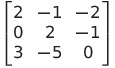Select Page

# Solutions for MCQ CBSE 12 Science Maths Continuity and Differentiability in English

Solutions for MCQ CBSE 12 Science Maths Continuity and Differentiability in English to enable students to get Solutions in a narrative video format for the specific question.

Expert Teacher provides Solutions for MCQ CBSE 12 Science Maths Continuity and Differentiability through Video Solutions in English language. This video solution will be useful for students to understand how to write an answer in exam in order to score more marks. This teacher uses a narrative style for a question from Continuity and Differentiability not only to explain the proper method of answering question, but deriving right answer too.

Please find the question below and view the Solution in a narrative video format.

Question:

Solution Video in English:

You can select video Solutions from other languages also. Please check Solutions in ( Hindi )

## Similar Questions from CBSE, 12th Science, Maths, Continuity and Differentiability

Question 1 : Differentiate the functionwith respect to x. (View Answer Video)

Question 2 : Differentiate w.r.t.x the function, for some fixed a > 0 and x > 0. (View Answer Video)

Question 3 : Differentiate the functionwith respect to x. (View Answer Video)

Question 4 : If x and y are connected parametrically by the equation, without eliminating the parameter, find. (View Answer Video)

Question 5 : Differentiate the function w.r.t.x. (View Answer Video)

### Inverse Trigonometric Functions

Question 1 : Write the principal value of(View Answer Video)

Question 2 : Write the value of(View Answer Video)

Question 3 :is equal to: (View Answer Video)

Question 4 :

Write the value of the following:Question 5 : Solve the equation :(View Answer Video)

### Matrices

Question 1 : Find the value of x from the equation:. (View Answer Video)

Question 2 : Given,, find the value of z. (View Answer Video)

Question 3 : Find the transpose of the matrix:. (View Answer Video)

Question 4 : Compute:. (View Answer Video)

Question 5 : Find the value of x, if. (View Answer Video)

### Determinant

Question 1 : Find the values of x, if. (View Answer Video)

Question 2 :  Find the values of x, if. (View Answer Video)

Question 3 : Let, where. THhen (View Answer Video)

Question 4 :  Evaluate the determinants:. (View Answer Video)

Question 5 : Evaluate the determinant:. (View Answer Video)